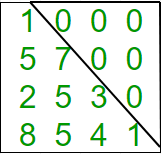GeeksforGeeks App
Open AppBrowser
Continue

# Java Program to check if matrix is lower triangular

Given a square matrix and the task is to check the matrix is in lower triangular form or not. A square matrix is called lower triangular if all the entries above the main diagonal are zero.Examples:

```Input : mat = {{1, 0, 0, 0},
{1, 4, 0, 0},
{4, 6, 2, 0},
{0, 4, 7, 6}};
Output : Matrix is in lower triangular form.

Input : mat = {{1, 0, 0, 0},
{4, 3, 0, 1},
{7, 9, 2, 0},
{8, 5, 3, 6}};
Output : Matrix is not in lower triangular form.```

## Java

 `// Java Program to check for``// a lower triangular matrix.``import` `java.io.*;` `class` `Lower_triangular``{``    ``int` `N = ``4``;` `    ``// Function to check matrix is``    ``// in lower triangular form or not.``    ``boolean` `isLowerTriangularMatrix(``int` `mat[][])``    ``{``        ``for` `(``int` `i = ``0``; i < N; i++)``            ``for` `(``int` `j = i + ``1``; j < N; j++)``                ``if` `(mat[i][j] != ``0``)``                    ``return` `false``;` `        ``return` `true``;``    ``}` `    ``// Driver function.``    ``public` `static` `void` `main(String args[])``    ``{``        ``Lower_triangular ob = ``new` `Lower_triangular();``        ``int` `mat[][] = { { ``1``, ``0``, ``0``, ``0` `},``                        ``{ ``1``, ``4``, ``0``, ``0` `},``                        ``{ ``4``, ``6``, ``2``, ``0` `},``                        ``{ ``0``, ``4``, ``7``, ``6` `} };``                        ` `        ``// Function call``        ``if` `(ob.isLowerTriangularMatrix(mat))``            ``System.out.println(``"Yes"``);``        ``else``            ``System.out.println(``"No"``);``    ``}``}` `// This code is contributed by Anshika Goyal.`

Output:

`Yes`

Time Complexity: O(n2), where n represents the number of rows and columns of the matrix.
Auxiliary Space: O(1), no extra space is required, so it is a constant.

Please refer complete article on Program to check if matrix is lower triangular for more details!

My Personal Notes arrow_drop_up